Thank you for visiting nature.com. You are using a browser version with limited support for CSS. To obtain the best experience, we recommend you use a more up to date browser (or turn off compatibility mode in Internet Explorer). In the meantime, to ensure continued support, we are displaying the site without styles and JavaScript.

# Emergence of coherence via transverse condensation in a uniform quasi-two-dimensional Bose gas

## Abstract

Phase transitions are ubiquitous in our three-dimensional world. By contrast, most conventional transitions do not occur in infinite uniform low-dimensional systems because of the increased role of thermal fluctuations. The crossover between these situations constitutes an important issue, dramatically illustrated by Bose-Einstein condensation: a gas strongly confined along one direction of space may condense along this direction without exhibiting true long-range order in the perpendicular plane. Here we explore transverse condensation for an atomic gas confined in a novel trapping geometry, with a flat in-plane bottom, and we relate it to the onset of an extended (yet of finite-range) in-plane coherence. By quench crossing the transition, we observe topological defects with a mean number satisfying the universal scaling law predicted by Kibble-Zurek mechanism. The approach described can be extended to investigate the topological phase transitions that take place in planar quantum fluids.

## Introduction

Bose–Einstein condensation (BEC) is a remarkably simple phase transition that can in principle occur in a fluid even in the absence of interatomic interactions. As a mere result of single-particle statistics, a phase-coherent fraction appears in the fluid, described by a uniform wave-function spanning the whole system. During the last two decades, cold atom experiments have been used to probe many aspects of BEC1,2,3. However, most of these cold atom studies are performed in the presence of a harmonic confinement. BEC becomes in this case a local transition: the condensate forms at the centre of the trap where the density is the largest, and interactions between particles play a dominant role in the equilibrium state of the fluid. In this geometry, the non-homogeneous character of the gas makes it difficult to address some important features of BEC, such as the existence of long-range phase coherence. The recent achievement of a three-dimensional (3D) Bose gas undergoing BEC in a box-like potential4,5 constitutes an important step forward, realizing the text-book paradigm of an extended and uniform coherent matter wave.

When turning to low-dimensional (low-D) systems, subtle effects emerge because of the entangled roles of Bose statistics and thermal fluctuations. First, in an infinite low-D ideal gas, no BEC is expected at non-zero temperature, because of the modification of the single-particle density of states with respect to the 3D case6. In other words, the phase coherence between two points tends to zero when their distance increases, contrary to the 3D situation. Second, Bose statistics can play a key role in the dimensional crossover between the 3D situation and a low-D one, by facilitating the freezing of some directions of space. Consider the uniform two-dimensional (2D) case obtained by imposing a tight harmonic trapping potential (frequency νz) along the third direction z. The transverse condensation phenomenon7,8,9 allows one to reach an effective 2D situation even in the ‘thermally unfrozen’ regime, where the quantum z is smaller than the thermal energy kBT (h and kB stand for Planck’s and Boltzmann’s constants). Third, for the 2D case in the presence of interactions between the particles, the situation gets more involved with the possibility of a superfluid, Berezinskii—Kosterlitz—Thouless (BKT) transition10,11 for a large enough phase-space-density, even though the absence of true long-range coherence remains valid12,13. This superfluid transition has been identified and characterized over the recent years with non-homogeneous, harmonically trapped Bose gases14,15,16,17,18,19,20.

Another key feature of phase transitions for uniform systems is the time needed to establish the coherence/quasi-coherence over the whole sample. As is well known for critical phenomena21, the coherence length and the thermalization time diverge at the transition point, thus limiting the size of the phase-coherent domains that are formed at its crossing. The Kibble-Zurek (KZ) theory22,23 allows one to evaluate the scaling of the domain size with the speed of the crossing. Once the transition has occurred, these domains start merging together. During this coarsening dynamics singularities taking the form of topological defects can be nucleated at their boundaries, with a spatial density directly related to the characteristic domain size. The KZ mechanism has been studied in a variety of experimental systems (see for example, refs 24, 25, 26, 27, 28, 29), including cold atomic gases30,31,32,33,34,35,36. In 2D quantum fluids, the singularities take the form of quantized vortices, that is, points of zero density around which the macroscopic wavefunction of the gas has a ±2π phase winding.

In this paper, we present an experimental realization of a uniform atomic Bose gas in a quasi-2D geometry, addressing both the steady state of the fluid and its quench dynamics. First with the gas in thermal equilibrium, we characterize the threshold for the emergence of an extended phase coherence by two independent methods, respectively, based on the measurement of the atomic velocity distribution and on matter–wave interferences. We show in particular that for the thermally unfrozen case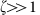(with ζ=kBT/z), the transverse condensation phenomenon induces an extended in-plane coherence. Second, we explore the quench dynamics of the gas prepared in an initial state such that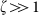and observe density holes associated to vortices. We study the relation between the cooling rate and the number of vortices that subsist after a given relaxation time, and we compare our results with the predictions of the KZ theory.

## Results

### Production of uniform gases in quasi-2D geometries

We prepare a cold 3D gas of rubidium (87Rb) atoms using standard laser and evaporative cooling techniques. Then we transfer the gas in a trap formed with two orthogonal laser beams at wavelength 532 nm, shorter than the atomic resonance wavelength (780 nm), so that the atoms are attracted towards the regions of low light intensity (Fig. 1a). The strong confinement along the z direction (vertical) is provided by a laser beam propagating along the x direction. It is prepared in a Hermite-Gauss mode, with a node in the plane z=0, and provides a harmonic confinement along the z direction with a frequency νz in the range 350–1,500 Hz. For the confinement in the horizontal xy plane, we realize a box-like potential by placing an intensity mask on the second laser beam path, propagating along the z direction (Fig. 1b). Depending on the study to be performed, we can vary the shape (disk, square, double rectangle) and the area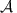(from 200 to 900 μm2) of the region accessible to the gas in the plane. The relevance of our system for the study of 2D physics is ensured by the fact that the size of the ground state along the z direction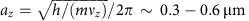is very small compared with the in-plane extension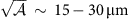. The number of atoms N that can be stored and reliably detected in this trap ranges between 1,000 and 100,000. We adjust the temperature of the gas in the interval T~10−250 nK by varying the intensity of the beam creating the box potential, taking advantage of evaporative cooling on the edges of this box. The ranges spanned by νz and T allow us to explore the dimensional crossover between the thermally frozen regime (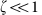) and the unfrozen one (). Examples of in situ images of 2D gases are shown in Fig. 1c–e.

### Phase coherence in 2D geometries

For an ideal gas, an important consequence of Bose-Einstein statistics is to increase the range of phase coherence with respect to the prediction of Boltzmann statistics. Here coherence is characterized by the one-body correlation function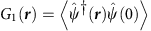, where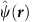[resp.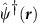] annihilates (resp. creates) a particle in r, and where the average is taken over the equilibrium state at temperature T. For a gas of particles of mass m described by Boltzmann statistics, G1(r) is a Gaussian function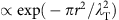, where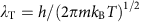is the thermal wavelength.

Consider the particular case of a 2D Bose gas (for example,). When its phase-space-density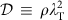becomes significantly larger than 1 (ρ stands for the 2D spatial density), the structure of G1(r) changes. In addition to the Gaussian function mentioned above, a broader feature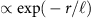develops, with the characteristic length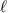that increases exponentially with (see ref. 37 and Supplementary Note 1)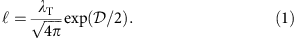Usually, two main effects amend this simple picture:

• In a finite system, when the predicted value ofbecomes comparable to the size L of the gas, one recovers a standard Bose-Einstein condensate, with a macroscopic occupation of the ground state of the box potential38. The G1 function then takes non-zero values for any rL and the phase coherence extends over the whole area of the gas.

• In the presence of weak repulsive interactions, the increase of the range of G1 for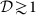is accompanied with a reduction of density fluctuations, with the formation of a ‘quasi-condensate’ or ‘pre-superfluid’ state15,16,39. This state is a medium that can support vortices, which will eventually pair at the superfluid BKT transition for a larger phase-space-density, around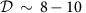for the present strength of interactions39. At the transition point, the coherence lengthdiverges and above this point, G1(r) decays algebraically.

### Role of the third dimension for in-plane phase coherence

When the thermal energy kBT is not negligibly small compared with the energy quantum z for the tightly confined dimension, the dynamics associated to this direction brings interesting novel features to the in-plane coherence. First, we note that the function G1(r) can be written in this case as a sum of contributions of the various states jz of the z motion (see Supplementary Note 1). The term with the longest range corresponds to the ground state jz=0, with an expression similar to equation (1), where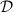is replaced by the phase-space-density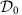associated to this state. Now, consider more specifically the unfrozen regime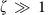. In this case, one expects that for very dilute gases only a small fraction f0 of the atoms occupies the jz=0 state; Boltzmann statistics indeed leads to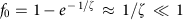. However, for large total phase-space densities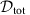, Bose-Einstein statistics modifies this result through the transverse condensation phenomenon (BEC)7: the phase-space-density that can be stored in the excited states jz≠0 is bounded from above, andcan thus become comparable to. This large value ofleads to a fast increase of the corresponding range of G1(r), thus linking the transverse condensation to an extended coherence in the xy plane. This effect plays a central role in our experimental investigation.

### Phase coherence revealed by velocity distribution imaging

To characterize the coherence of the gas, we study the velocity distribution, that is, the Fourier transform of the G1(r) function. We approach this velocity distribution in the xy plane by performing a 3D time-of-flight (3D ToF): we suddenly switch off the trapping potentials along the three directions of space, let the gas expand for a duration τ, and finally image the gas along the z axis. In such a 3D ToF, the gas first expands very fast along the initially strongly confined direction z. Thanks to this fast density drop, the interparticle interactions play nearly no role during the ToF and the slower evolution in the xy plane is governed essentially by the initial velocity distribution of the atoms. The time-of-flight (ToF) duration τ is chosen so that the size expected for a Boltzmann distribution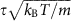is at least twice the initial extent of the cloud. Typical examples of ToF images are given in Fig. 2a–f. Whereas for the hottest and less dense configurations, the spatial distribution after ToF has a quasi-pure Gaussian shape, a clear non-Gaussian structure appears for larger N or smaller T. A sharp peak emerges at the centre of the cloud of the ToF picture, signalling an increased occupation of the low-momentum states with respect to Boltzmann statistics, or equivalently a coherence length significantly larger than λT.

In order to analyse this velocity distribution, we chose as a fit function the sum of two Gaussians of independent sizes and amplitudes, containing N1 and N2 atoms, respectively (see Fig. 2d–f). We consider the bimodality parameter Δ=N1/N defined as the ratio of the number of atoms N1 in the sharpest Gaussian to the total atom number N=N1+N2. A typical example for the variations of Δ with N at a given temperature is shown in Fig. 2g for an initial gas with a square shape (side length L=24 μm). It shows a sharp crossover, with essentially no bimodality (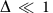) below a critical atom number Nc(T) and a fast increase of Δ for N>Nc(T). We extract the value Nc(T) by fitting the function Δ (1−(Nc/N)0.6) to the data. We chose this function as it provides a good representation of the predictions for an ideal Bose gas in similar conditions (see Methods).

### Phase coherence revealed by matter–wave interference

Matter–wave interferences between independent atomic or molecular clouds are a powerful tool to monitor the emergence of extended coherence4,14,40,41,42. To observe these interferences in our uniform setup, we first produced two independent gases of similar density and temperature confined in two coplanar parallel rectangles, separated by a distance of 4.5 μm along the x direction (see Fig. 1e). Then we suddenly released the box potential providing confinement in the xy plane, while keeping the confinement along the z direction (2D ToF). The latter point ensures that the atoms stay in focus with our imaging system, which allows us to observe interference fringes with a good resolution in the region where the two clouds overlap. A typical interference pattern is shown in Fig. 3a, where the fringes are (roughly) parallel to the y axis, and show some waviness that is linked to the initial phase fluctuations of the two interfering clouds.

We use these interference patterns to characterize quantitatively the level of coherence of the gases initially confined in the rectangles. For each line y of the pixelized image acquired on the CCD camera, we compute the x-Fourier transformof the spatial density ρ(x, y) (Fig. 3b). For a given y, this function is peaked at a momentum kp(y)>0 that may depend (weakly) on the line index y. Then we consider the function that characterizes the correlation of the complex fringe contrast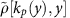along two lines separated by a distance d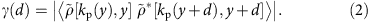Here * denotes the complex conjugation and the average is taken over the lines y that overlap with the initial rectangles. If the initial clouds were two infinite, parallel lines with the same G1(y), one would have γ(d)=|G1(d)|2 (ref. 43). Here the non-zero extension of the rectangles along x and their finite initial size along y make it more difficult to provide an analytic relation between γ and the initial G1(r) of the gases. However, γ(d) remains a useful and quantitative tool to characterize the fringe pattern. For a gas described by Boltzmann statistics, the width at 1/e of G1(r) is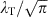and remains below 1 μm for the temperature range investigated in this work. Since we are interested in the emergence of coherence over a scale that significantly exceeds this value, we use the following average as a diagnosis tool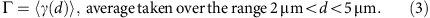For the parameter Γ to take a value significantly different from 0, one needs a relatively large contrast on each line, and relatively straight fringes over the relevant distances d, so that the phases of the different complex contrasts do not average out.

For a given temperature T, the variation of Γ with N shows the same threshold-type behaviour as the bimodality parameter Δ. One example is given in Fig. 3c, from which we infer the threshold value for the atom number Nc(T) needed to observe interference fringes with a significant contrast.

### Scaling laws for the emergence of coherence

We have plotted in Fig. 4 the ensemble of our results for the threshold value of the total 2D phase-space-density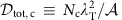as a function of ζ=kBT/z, determined both from the onset of bimodality as in Fig. 2g (closed symbols) or from the onset of visible interference as in Fig. 3c (open symbols). Two trapping configurations have been used along the z direction, νz=1,460 Hz and νz=365 Hz. In the first case, the z direction is nearly frozen for the temperatures studied here (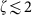). In the second one, the z direction is thermally unfrozen (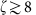). All points approximately fall on a common curve, independent of the shape and the size of the gas: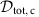varies approximately linearly with ζ with the fitted slope 1.4 (3) forand approaches a finite value ~4 for.Figure 4: Scaling law for the emergence of coherence in a uniform Bose gas in a quasi-2D geometry.

In the frozen case, a majority of atoms occupy the vibrational ground state jz=0 of the motion along the z direction, so thatessentially represents the 2D phase-space-density associated to this single transverse quantum state. Then for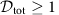, we know from equation (1) and the associated discussion that a broad component arises in G1 with a characteristic lengththat increases exponentially with the phase-space-density. The observed onset of extended coherence around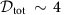can be understood as the place wherestarts to exceed significantly λT. The regime aroundis reminiscent of the presuperfluid state identified in refs 15, 16. It is different from the truly superfluid phase, which is expected at a higher phase-space-density (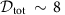) for our parameters39. Therefore, the thresholdis not associated to a true phase transition, but to a crossover where the spatial coherence of the gas increases rapidly with the control parameter N.

For νz=365 Hz, the gas is in the ‘unfrozen regime’ (), which could be naively thought as irrelevant for 2D physics since according to Boltzmann statistics, many vibrational states along z should be significantly populated. However, thanks to the BEC phenomenon presented above, a macroscopic fraction of the atoms can accumulate in the jz=0 state. This happens when the total phase-space-density exceeds the threshold for BEC (cf. Supplementary Note 1 and Supplementary Fig. 1):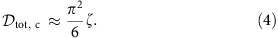In the limit ζ→∞, BEC corresponds to a phase transition of the same nature as the ideal gas BEC in 3D (see Supplementary Note 1 and Supplementary Fig. 2). In the present context of our work, we emphasize that although BEC originates from the saturation of the occupation of the excited states along z, it also affects the coherence properties of the gas in the xy plane. In particular whenrises from 0 to, the coherence length in xy increases from ~λT (the non-degenerate result) to ~az, the size of the ground state of the z motion (see Supplementary Note 1, Supplementary Fig. 3 and Supplementary Table 1). This increase can be interpreted by noting that when BEC occurs (equation 4), the 3D spatial density in the central plane (z=0) is equal to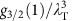, where gs is the polylogarithm of order s and g3/2(1)≈2.612 (see Supplementary Note 1 and Supplementary Fig. 4). For an infinite uniform 3D Bose gas with this density, a true BEC occurs and the coherence length diverges. Because of the confinement along the z direction, such a divergence cannot occur in the present quasi-2D case. Instead, the coherence length along z is by essence limited to the size az of the jz=0 state. When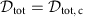the same limitation applies in the transverse plane, giving rise to coherence volumes that are grossly speaking isotropic. Whenis increased further, the coherence length in the xy plane increases, while remaining limited to az along the z direction. The results shown in Fig. 4 are in line with this reasoning. For, the emergence of coherence in the xy plane occurs for a total phase-space-density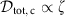, with a proportionality coefficient α=1.4(3) in good agreement with the prediction π2/6≈1.6 of equation (4).

We have also plotted in Fig. 4 contour lines characterizing the coherence range in terms of ζ and. Using ideal Bose gas theory, we calculated the one-body coherence function G1(r) and determined the distance rf over which it decreases by a given factor f with respect to G1(0). We chose the value f=20 to explore the long tail that develops in G1 when phase coherence emerges. The contour lines shown in Fig. 4 correspond to given values of r20/λT; they should not be considered as fits to the data, but as an indication of a coherence significantly larger than the one obtained from Boltzmann statistics (for which r20λT). The fact that the threshold phase-space densitiesfollow quite accurately these contour lines validates the choice of tools (non-Gaussian velocity distributions, matter–wave interferences) to characterize the onset of coherence.

### Observation of topological defects

From now on we use the weak trap along z (νz=365 Hz) so that the onset of extended coherence is obtained thanks to the transverse condensation phenomenon. We are interested in the regime of strongly degenerate, interacting gases, which is obtained by pushing the evaporation down to a point where the residual thermal energy kBT becomes lower than the chemical potential μ (see Methods for the calculation of μ in this regime). The final box potential is ~kB × 40 nK, leading to an estimated temperature of ~10 nK, whereas the final density (~50 μm−2) leads to μ≈kB × 14 nK. In these conditions, for most realizations of the experiment, defects are present in the gas. They appear as randomly located density holes after a short 3D ToF (Fig. 5a,b), with a number fluctuating between 0 and 5. To identify the nature of these defects, we have performed a statistical analysis of their size and contrast, as a function of their location and of the ToF duration τ (Fig. 5c,d). For a given τ, all observed holes have similar sizes and contrasts. The core size increases approximately linearly with τ, with a nearly 100% contrast. This favours the interpretation of these density holes as single vortices, for which the 2π phase winding around the core provides a topological protection during the ToF. This would be the case neither for vortex–antivortex pairs nor phonons, for which one would expect large fluctuations in the defect sizes and lower contrasts.

### Dynamical origin of the topological defects

In principle, the vortices observed in the gas could be due to steady-state thermal fluctuations. BKT theory indeed predicts that vortices should be present in an interacting 2D Bose gas around the superfluid transition point11. Such ‘thermal’ vortices have been observed in non-homogeneous atomic gases, either interferometrically14 or as density holes in the trap region corresponding to the critical region20. However, for the large and uniform phase-space densities that we obtain at the end of the cooling process (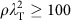), ref. 44 predicts a vanishingly small probability of occurrence for such thermal excitations. This supports a dynamical origin for the observed defects.

To investigate further this interpretation, we can vary the two times that characterize the evolution of the gas, the duration of evaporation tevap and the hold duration after evaporation thold (see Fig. 1a). For the results presented in this section, we fixed thold=500 ms and studied the evolution of the average vortex number Nv as a function of tevap. The corresponding data, given in Fig. 6a, show a decrease of Nv with tevap, passing from Nv≈1 for tevap=50 ms to Nv≈0.3 for tevap=250 ms. For longer evaporation times, Nv remains approximately constant around 0.35(5).

The decrease of Nv with tevap suggests that the observed vortices are nucleated via a KZ type mechanism22,23,45, occurring when the transition to the phase coherent regime is crossed. However, applying the KZ formalism to our setup is not straightforward. In a weakly interacting, homogeneous 3D Bose gas, BEC occurs when the 3D phase-space-density reaches the critical value g3/2(1). For our quasi-2D geometry, transverse condensation occurs when the 3D phase-space-density in the central plane z=0 reaches this value. At the transition point, the KZ formalism relates the size of phase-coherent domains to the cooling speed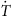. For fast cooling, KZ theory predicts domain sizes for a 3D fluid that are smaller than or comparable to the thickness az of the lowest vibrational state along z; it can thus provide a good description of our system. For a slower cooling, coherent domains much larger than az would be expected in 3D at the transition point. The 2D nature of our gas leads in this case to a reduction of the in-plane correlation length. In the slow cooling regime, we thus expect to find an excess of topological defects with respect to the KZ prediction for standard 3D BEC.

More explicitly we expect for fast cooling, hence short tevap, a power-law decay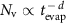with an exponent d given by the KZ formalism for 3D BEC. The fit of this function to the measured variation of Nv for tevap≤250 ms leads to d=0.69(17) (see Fig. 6a). This is in good agreement with the prediction d=2/3 obtained from the critical exponents of the so-called ‘F model’21, which is believed to describe the universality class of the 3D BEC phenomenon. For comparison, the prediction for a pure mean-field transition, d=1/2, is notably lower than our result.

For longer tevap, the above described excess of vortices due to the quasi-2D geometry should translate in a weakening of the decrease of Nv with tevap. The non-zero plateau observed in Fig. 6a for tevap≥250 ms may be the signature of such a weakening. Other mechanisms could also play a role in the nucleation of vortices for slow cooling. For example, because of the box potential residual rugosity, the gas could condense into several independent patches of fixed geometry, which would merge later during the evaporation ramp and stochastically form vortices with a constant probability.

### Lifetime of the topological defects

The variation of the number of vortices Nv with the hold time thold allows one to study the fate of vortices that have been nucleated during the evaporation. We show in Fig. 6b the results obtained when fixing the evaporation to a short value tevap=50 ms. We observe a decay of Nv with the hold time, from Nv=2.3 initially to 0.3 at long thold (2 s). To interpret this decay, we modelled the dynamics of the vortices in the gas with two ingredients: first the conservative motion of a vortex in the velocity field created by the other vortices, including the vortex images from the boundaries of the box potential46; second the dissipation induced by the scattering of thermal excitations by the vortices, which we describe phenomenologically by a friction force that is proportional to the non-superfluid fraction of atoms in the gas47. During this motion, a vortex annihilates when it reaches the edge of the trap or encounters another vortex of opposite charge. The numerical solution of this model leads to a non-exponential decay of the average number of vortices, with details that depend on the initial number of vortices and their locations.

Assuming a uniform random distribution of vortices at the end of the evaporation, we have compared the predictions of this model to our data. It gives the following values of the two adjustable parameters of the model, the initial number of vortices Nv,0=2.5(2) and the superfluid fraction 0.94(2); the corresponding prediction is plotted as a continuous line in Fig. 6b. We note that at short thold, the images of the clouds are quite fuzzy, probably because of non-thermal phononic excitations produced (in addition to vortices) by the evaporation ramp. The difficulty to precisely count vortices in this case leads to fluctuations of Nv at short thold as visible in Fig. 6b. The choice thold=500 ms in Fig. 6a was made accordingly.

The finite lifetime of the vortices in our sample points to a general issue that one faces in the experimental studies on the KZ mechanism. In principle, the KZ formalism gives a prediction on the state of the system just after crossing the critical point. Experimentally we observe the system at a later stage, at a moment when the various domains have merged, and we detect the topological defects formed from this merging. In spite of their robustness, the number of vortices is not strictly conserved after the crossing of the transition and its decrease depends on their initial positions. A precise comparison between our results and KZ theory should take this evolution into account, for example, using stochastic mean-field methods48,49,50,51.

## Discussion

Using a box-like potential created by light, we developed a setup that allowed us to investigate the quantum properties of atomic gases in a uniform quasi-2D configuration. Thanks to the precise control of atom number and temperature, we characterized the regime for which phase coherence emerges in the fluid. The uniform character of the gas allowed us to disentangle the effects of ideal gas statistics for in-plane motion, the notion of transverse condensation along the strongly confined direction and the role of interactions. This is to be contrasted with previous studies that were performed in the presence of a harmonic confinement in the plane, where these different phenomena could be simultaneously present in the non-homogeneous atomic cloud.

For the case of a weakly interacting gas considered here, our observations highlight the importance of Bose statistics in the emergence of extended phase coherence. This coherence is already significant for phase-space densities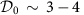, well below the value required either for the superfluid BKT transition or for the full BEC in the ground state of the box (see Supplementary Note 2 and Supplementary Fig. 5). For our parameters, the latter transitions are expected around the same phase-space-density (~8–10) meaning that when the superfluid criterion is met, the coherence length set by Bose statistics is comparable to the box size.

By cooling the gas further, we entered the regime where interactions dominate over thermal fluctuations. This allowed us to visualize with a very good contrast the topological defects (vortices) that are created during the formation of the macroscopic matter-wave, as a result of a KZ type mechanism. Here we focused on the relation between the vortex number and the cooling rate. Further investigations could include correlation studies on vortex positions, which can shed light on their nucleation process and their subsequent evolution52.

Our work motivates future research in the direction of strongly interacting 2D gases19, for which the order of the various transitions could be interchanged. In particular, the criticalfor the BKT transition should decrease, and reach ultimately the universal value of the ‘superfluid jump’,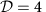(ref. 53). In this case, the emergence of extended coherence in the 2D gas would be essentially driven by the interactions. Indeed, once the superfluid transition is crossed, the one-body correlation function is expected to decay very slowly, G1(r) rα, with α<1/4. It would be interesting to revisit the statistics of formation of quench-induced topological defects in this case, for which significant deviations to the KZ power-law scaling have been predicted54,55.

## Methods

### Characterization of the box-like potential

We create the box-like potential in the xy plane using a laser beam that is blue-detuned with respect to the 87Rb resonance. At the position of the atomic sample, we image a dark mask placed on the path of the laser beam. This mask is realized by a metallic deposit on a wedged, anti-reflective-coated glass plate. We characterize the box-like character of the resulting trap in two ways. First, the flatness of the domain where the atoms are confined is characterized by the root mean square intensity fluctuations of the inner dark region of the beam profile. The resulting variations of the dipolar potential are δU/Ubox~3%, where Ubox is the potential height on the edges of the box. The ratio δU/kBT varies from ~40% at the loading temperature to ~10% at the end of the evaporative cooling (ramp of Ubox, see below). In particular, it is of ~20% at the transverse condensation point for the configuration in which the vortex data have been acquired. Second, the sharp spatial variation of the potential at the edges of the box-like trapping region is characterized by the exponent α of a power-law fit U(r) rα along with a radial cut. We restrict the fitting domain to the central region where U(r)<Ubox/4 and find α~10–15, depending on the size and the shape of the box.

### Imaging of the atomic density distribution

We measure the atomic density distribution in the xy plane using resonant absorption imaging along z. We use two complementary values for the probe beam intensity I. First, we use a conventional low-intensity technique with I/Isat≈0.7, where Isat is the saturation intensity of the Rb resonance line, with a probe pulse duration of 20 μs. This procedure enables a reliable detection of low-density atomic clouds, but it is unfaithful for high-density ones, especially in the 2D geometry because of multiple scattering effects between neighbouring atoms56. We thus complement it by a high-intensity technique inspired from ref. 57, in which we apply a short pulse of 4 μs of an intense probe beam with I/Isat≈40. ToF bimodality measurements (where the cloud is essentially 3D at the moment of detection) were performed with the low-intensity procedure. This was also the case for the matter–wave interference measurements, for which we reached a better fringe visibility in this case. In situ images in Fig. 1 and all data related to vortices (for example, Fig. 5a,b) in the strongly degenerate gases were taken with high-intensity imaging. We estimate the uncertainty on the atom number to be of 20%.

### Ideal gas description of trapped atomic samples

We consider a gas of N non-interacting bosonic particles confined in a square box of size L in the xy plane, and in a harmonic potential well of frequency νz along z. The eigenstates of the single-particle Hamiltonian are labelled by three integers jx, jy≥1, jz≥0:where az=(h/z)1/2/(2π) and χj is the normalized jth Hermite function. Their energies and occupation factors are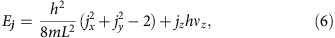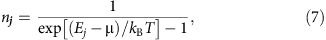where μ<0 is the chemical potential of the gas and N=∑jnj. The average value of any one-body observable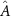can then be calculated: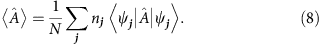### Interaction energy estimate for weakly interacting gases

We estimate the local value of the interaction energy per particle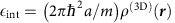, where a=5.1 nm is the 3D scattering length characterizing s-wave interactions for 87Rb atoms and ρ(3D)(r) the spatial 3D density estimated using the ideal gas description. It is maximal at trap centre r=0. For example, using a typical experimental condition with N=40,000 atoms in a square box of size L=24 μm at T=200 nK, we find a maximal 3D density of ρ(3D)(0)=13.8 μm−3. The mean-field interaction energy for an atom localized at the centre of cloud is then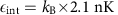. We note that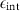is negligible compared with kBT and z for all atomic configurations corresponding to the onset of an extended phase coherence. In this case, the interactions play a negligible role in the 2D ToF expansion that we use to reveal matter–wave interferences.

### Temperature calibration

All temperatures indicated in the paper are deduced from the value of the box potential, assuming that the evaporation barrier provided by Ubox sets the thermal equilibrium state of the gas. This hypothesis was tested, and the relation between T and Ubox calibrated, using atomic assemblies with a negligible interaction energy. For these assemblies, we compared the variance of their velocity distribution Δv2 obtained from a ToF measurement to the prediction of equation (8). The calibration obtained from this set of measurements can be empirically written as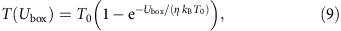where the values of the dimensionless parameter η and of the reference temperature T0 slightly depend on the precise shape of the trap. For the square trap of side 24 μm, we obtain T0=191(6) nK and η=3.5(3). The reason for which T saturates when the box potential increases to infinity is due to the residual evaporation along the vertical direction, above the barrier created by the horizontal Hermite–Gauss beam.

### Power exponent for fitting Nc

We estimate the behaviour of Δ(N) at fixed T using Bose law for a ideal gas. We compute from equation (8) the equilibrium velocity distribution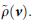Then we estimate the spatial density after a ToF of duration τ (for a disk trap of radius R) via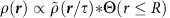, where stands for the convolution operator and Θ for the Heaviside function. We fit ρ(r) to a double Gaussian and compute the atom fraction in the sharpest Gaussian Δ, similar to the processing of experimental data. To simulate our experimental results, we consider νz=350 Hz, R=12 μm, τ=14 ms and T varying from 100 to 250 nK. For a given T, we record Δ while varying the total atom number N from 0.06 to 4 times the theoretical critical number for(see Supplementary Note 1). We fit Δ(N) between Nmin=0.06 Nc,th and a varying Nmax in 1.1–4 Nc,th, to f(N)=(1−(Nc/N)α) with Nc as a free parameter and a fixed α. For all considered T and Nmax, choosing α=0.6 provides both a good estimate of Nc (between 0.93 and 0.99 Nc,th) and a satisfactory fit (average coefficient of determination 0.94).

### Chemical potential in the degenerate interacting regime

To compute the chemical potential μ of highly degenerate interacting gases, we perform a T=0 mean-field analysis. We solve numerically the 3D Gross–Pitaevskii equation in imaginary time using a split-step method, and we obtain the macroscopic ground-state wave-function ψ(r). Then we calculate the different energy contributions at T=0—namely the potential energy Epot, the kinetic energy Ekin and interaction energy Eint—by integrating over space: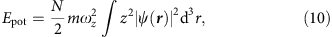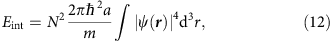with ωz=2πνz. We obtain the value of the chemical potential μ by taking the derivative of the total energy with respect to N and subtracting the single-particle ground-state energy: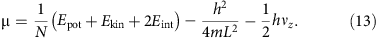In the numerical calculation, we typically use time steps of 10−4 ms and compute the evolution for 10 ms. The 3D grid contains 152 × 152 × 32 voxels, with a voxel size 0.52 × 0.52 × 0.26 μm3.

### Analysis of the density holes created by the vortices

We first calculate the normalized density profile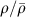, where the average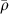is taken over the set of images with the same ToF duration τ. Then we look for density minima with a significant contrast and size. Finally, for each significant density hole, we select a square region centred on it with a size that is approximately three times larger than the average hole size for this τ. In this region, we fit the function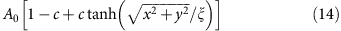to the normalized density profile, where A0 accounts for density fluctuations. We also correct for imaging imperfections (finite imaging resolution and finite depth of field) by performing a convolution of the function defined in equation (14) by a Gaussian of width 1 μm, which we determined from a preliminary analysis.

How to cite this article: Chomaz, L. et al. Emergence of coherence via transverse condensation in a uniform quasi-two-dimensional Bose gas. Nat. Commun. 6:6162 doi: 10.1038/ncomms7162 (2015).

## References

1. 1

Pethick, C. & Smith, H. Bose-Einstein Condensation in Dilute Gases Cambridge Univ. (2002).

2. 2

Pitaevskii, L. & Stringari, S. Bose-Einstein Condensation Oxford Univ. (2003).

3. 3

Leggett, A. J. Quantum Liquids Oxford Univ. (2006).

4. 4

Gaunt, A. L., Schmidutz, T. F., Gotlibovych, I., Smith, R. P. & Hadzibabic, Z. Bose–Einstein condensation of atoms in a uniform potential. Phys. Rev. Lett. 110, 200406 (2013).

5. 5

Gotlibovych, I. et al. Observing properties ofan interacting homogeneous Bose-Einstein condensate: Heisenberg-limited momentum spread, interaction energy, and free-expansion dynamics. Phys. Rev. A 89, 061604 (2014).

6. 6

Huang, K. Statistical Mechanics Wiley (1987).

7. 7

van Druten, N. J. & Ketterle, W. Two-step condensation of the ideal Bose gas in highly anisotropic traps. Phys. Rev. Lett. 79, 549–552 (1997).

8. 8

Armijo, J., Jacqmin, T., Kheruntsyan, K. & Bouchoule, I. Mapping out the quasicondensate transition through the dimensional crossover from one to three dimensions. Phys. Rev. A 83, 021605 (2011).

9. 9

RuGway, W. et al. Observation of transverse Bose-Einstein Condensation via Hanbury Brown-Twiss Correlations. Phys. Rev. Lett. 111, 093601 (2013).

10. 10

Berezinskii, V. L. Destruction of long-range order in one-dimensional and two-dimensional system possessing a continuous symmetry group - II. quantum systems. Soviet Phys. JETP 34, 610 (1971).

11. 11

Kosterlitz, J. M. & Thouless, D. J. Ordering, metastability and phase transitions in two-dimensional systems. J. Phys. C Solid State Phys. 6, 1181 (1973).

12. 12

Mermin, N. D. & Wagner, H. Absence of ferromagnetism or antiferromagnetism in one- or two-dimensional isotropic Heisenberg models. Phys. Rev. Lett. 17, 1133 (1966).

13. 13

Hohenberg, P. C. Existence of long-range order in one and two dimensions. Phys. Rev 158, 383 (1967).

14. 14

Hadzibabic, Z., Krüger, P., Cheneau, M., Battelier, B. & Dalibard, J. Berezinskii-Kosterlitz-Thouless crossover in a trapped atomic gas. Nature 441, 1118–1121 (2006).

15. 15

Cladeé, P., Ryu, C., Ramanathan, A., Helmerson, K. & Phillips, W. D. Observation of a 2D Bose gas: from thermal to quasicondensate to superfluid. Phys. Rev. Lett. 102, 170401 (2009).

16. 16

Tung, S., Lamporesi, G., Lobser, D., Xia, L. & Cornell, E. A. Observation of the presuperfluid regime in a two-dimensional Bose gas. Phys. Rev. Lett. 105, 230408 (2010).

17. 17

Hung, C.-L., Zhang, X., Gemelke, N. & Chin, C. Observation of scale invariance and universality in two-dimensional Bose gases. Nature 470, 236 (2011).

18. 18

Desbuquois, R. et al. Superfluid behaviour of a two-dimensional Bose gas. Nat. Phys. 8, 645–648 (2012).

19. 19

Ha, L.-C. et al. Strongly interacting two-dimensional Bose gases. Phys. Rev. Lett. 110, 145302 (2013).

20. 20

Choi, J.-y., Seo, S. W. & Shin, Y.-i. Observation of thermally activated vortex pairs in a quasi-2D Bose gas. Phys. Rev. Lett. 110, 175302 (2013).

21. 21

Hohenberg, P. C. & Halperin, B. I. Theory of dynamic critical phenomena. Rev. Modern Phys. 49, 435 (1977).

22. 22

Kibble, T. W. Topology of cosmic domains and strings. J. Phys. A Math. Gen. 9, 1387 (1976).

23. 23

Zurek, W. Cosmological experiments in superfluid helium? Nature 317, 505–508 (1985).

24. 24

Chuang, I., Durrer, R., Turok, N. & Yurke, B. Cosmology in the laboratory: defect dynamics in liquid crystals. Science 251, 1336–1342 (1991).

25. 25

Ruutu, V. et al. Vortex formation in neutron-irradiated superfluid 3He as an analogue of cosmological defect formation. Nature 382, 334–336 (1996).

26. 26

Bauerle, C., Bunkov, Y. M., Fisher, S., Godfrin, H. & Pickett, G. Laboratory simulation of cosmic string formation in the early Universe using superfluid 3 He. Nature 382, 332–334 (1996).

27. 27

Ulm, S. et al. Observation of the Kibble-Zurek scaling law for defect formation in ion crystals. Nat. Commun. 4, 2290 (2013).

28. 28

Pyka, K. et al. Topological defect formation and spontaneous symmetry breaking in ion Coulomb crystals. Nat. Commun. 4, 2291 (2013).

29. 29

Monaco, R., Mygind, J., Rivers, R. & Koshelets, V. Spontaneous fluxoid formation in superconducting loops. Phys. Rev. B 80, 180501 (2009).

30. 30

Sadler, L. E., Higbie, J. M., Leslie, S. R., Vengalattore, M. & Stamper-Kurn, D. M. Spontaneous symmetry breaking in a quenched ferromagnetic spinor Bose-Einstein condensate. Nature 443, 312–315 (2006).

31. 31

Weiler, C. N. et al. Spontaneous vortices in the formation of Bose-Einstein condensates. Nature 455, 948–951 (2008).

32. 32

Chen, D., White, M., Borries, C. & DeMarco, B. Quantum quench of an atomic mott insulator. Phys. Rev. Lett. 106, 235304 (2011).

33. 33

Lamporesi, G., Donadello, S., Serafini, S., Dalfovo, F. & Ferrari, G. Spontaneous creation of Kibble-Zurek solitons in a Bose-Einstein condensate. Nat. Phys. 9, 656–660 (2013).

34. 34

Braun, S. et al. Emergence of coherence and the dynamics of quantum phase transitions. Preprint at http://arxiv.org/abs/1403.7199 (2014).

35. 35

Corman, L. et al. Quench-induced supercurrents in an annular Bose gas. Phys. Rev. Lett. 113, 135302 (2014).

36. 36

Navon, N., Gaunt, A. L, Smith, R. P. & Hadzibabic, Z. Critical dynamics of spontaneous symmetry breaking in a homogeneous Bose gas. Preprint at http://arxiv.org/abs/1410.8487 (2014).

37. 37

Hadzibabic, Z. & Dalibard, J. Two-dimensional Bose fluids: An atomic physics perspective. Rivista del Nuovo Cimento 34, 389 (2011).

38. 38

Petrov, D. S., Holzmann, M. & Shlyapnikov, G. V. Bose-Einstein condensation in quasi-2D trapped gases. Phys. Rev. Lett. 84, 2551 (2000).

39. 39

Prokof'ev, N. V. & Svistunov, B. V. Two-dimensional weakly interacting Bose gas in the fluctuation region. Phys. Rev. A 66, 043608 (2002).

40. 40

Andrews, M. R. et al. Observation ofinterference between two Bose condensates. Science 275, 637 (1997).

41. 41

Hofferberth, S., Lesanovsky, I., Fischer, B., Schumm, T. & Schmiedmayer, J. Non-equilibrium coherence dynamics in one-dimensional Bose gases. Nature 449, 324–327 (2007).

42. 42

Kohstall, C. et al. Observation of interference between two molecular Bose-Einstein condensates. New J. Phys. 13, 065027 (2011).

43. 43

Polkovnikov, A., Altman, E. & Demler, E. Interference between independent fluctuating condensates. Proc. Natl Acad. Sci. USA 103, 6125 (2006).

44. 44

Giorgetti, L., Carusotto, I. & Castin, Y. Semiclassical field method for the equilibrium Bose gas and application to thermal vortices in two dimensions. Phys. Rev. A 76, 013613 (2007).

45. 45

Anglin, J. R. & Zurek, W. H. Vortices in the wake of rapid Bose-Einstein condensation". Phys. Rev. Lett. 83, 1707 (1999).

46. 46

Donnelly, R. J. Quantized Vortices in Helium II Cambridge Univ. (1991).

47. 47

Fedichev, P. O. & Shlyapnikov, G. V. Dissipative dynamics of a vortex state in a trapped Bose-condensed gas. Phys. Rev. A 60, R1779–R1782 (1999).

48. 48

Bisset, R. N., Davis, M. J., Simula, T. P. & Blakie, P. B. Quasicondensation and coherence in the quasi-two-dimensional trapped Bose gas. Phys. Rev. A 79, 033626 (2009).

49. 49

Mathey, L. & Polkovnikov, A. Light cone dynamics and reverse Kibble-Zurek mechanism in two-dimensional superfluids following a quantum quench. Phys. Rev. A 81, 033605 (2010).

50. 50

Das, A., Sabbatini, J. & Zurek, W. H. Winding up superfluid in a torus via Bose Einstein condensation. Sci. Rep. 2, 352 (2012).

51. 51

Cockburn, S. P. & Proukakis, N. P. Ab initio methods for finite-temperature two-dimensional Bose gases. Phys. Rev. A 86, 033610 (2012).

52. 52

Freilich, D. V., Bianchi, D. M., Kaufman, A. M., Langin, T. K. & Hall, D. S. Real-time dynamics of single vortex lines and vortex dipoles in a Bose–Einstein condensate. Science 329, 1182–1185 (2010).

53. 53

Nelson, D. R. & Kosterlitz, J. M. Universal jump in the superfluid density of two-dimensional superfluids. Phys. Rev. Lett. 39, 1201 (1977).

54. 54

Jelicé, A. & Cugliandolo, L. F. Quench dynamics of the 2d XY model. J. Stat. Mech. Theory Exp. 2011, P02032 (2011).

55. 55

Dziarmaga, J. & Zurek, W. H. Quench in 1D Bose-Hubbard model: topological defects and excitations from Kosterlitz-Thouless phase transition dynamics. Sci. Rep 4, 5950 (2014).

56. 56

Chomaz, L., Corman, L., Yefsah, T., Desbuquois, R. & Dalibard, J. Absorption imaging of a quasi-two-dimensional gas: a multiple scattering analysis. New J. Phys. 14, 055001 (2012).

57. 57

Reinaudi, G., Lahaye, T., Wang, Z. & Guery-Odelin, D. Strong saturation absorption imaging of dense clouds ofultracold atoms. Opt. Lett. 32, 3143 (2007).

58. 58

Ketterle, W. & Van Druten, N. Evaporative cooling of trapped atoms. Adv. Atomic Mol. Ppt. Phys. 37, 181–236 (1996).

## Acknowledgements

We thank J. Palomo and D. Perconte for the realization of the intensity masks and Z. Hadzibabic for several useful discussions. This work is supported by the IFRAF, ANR (ANR-12- 247 BLANAGAFON), ERC (Synergy grant UQUAM) and the Excellence Cluster CUI. L.Ch. and L.Co. acknowledge the support from the DGA, and C.W. acknowledges the support from the EU (PIEF-GA-2011- 299731).

## Author information

Authors

### Contributions

L.Ch., L.Co. and T.B. performed the experiment and carried out the preliminary data analysis, with contributions from J.B., S.N. and J.D.; R.D. and C.W. participated in the preparation of the experimental setup. L.Ch. performed the detailed data analysis and their modelling. S.N. developed the simulation on vortex dynamics. L.Ch. and J.D. wrote the manuscript with contributions from all authors.

### Corresponding author

Correspondence to Lauriane Chomaz.

## Ethics declarations

### Competing interests

The authors declare no competing financial interests.

## Supplementary information

### Supplementary Information

Supplementary Figures 1-5, Supplementary Table 1, Supplementary Notes 1-2 and Supplementary References. (PDF 519 kb)

## Rights and permissions

Reprints and Permissions

• ### Tan’s two-body contact across the superfluid transition of a planar Bose gas

• Y.-Q. Zou
• , B. Bakkali-Hassani
• , C. Maury
• , É. Le Cerf
• , S. Nascimbene
• , J. Dalibard
•  & J. Beugnon

Nature Communications (2021)

• ### Phase coherence in out-of-equilibrium supersolid states of ultracold dipolar atoms

• P. Ilzhöfer
• , M. Sohmen
• , G. Durastante
• , C. Politi
• , A. Trautmann
• , G. Natale
• , G. Morpurgo
• , T. Giamarchi
• , L. Chomaz
• , M. J. Mark
•  & F. Ferlaino

Nature Physics (2021)

• ### Berezinskii–Kosterlitz–Thouless phase induced by dissipating quasisolitons

• Krzysztof Gawryluk
•  & Mirosław Brewczyk

Scientific Reports (2021)

• ### Observation of first and second sound in a BKT superfluid

• Panagiotis Christodoulou
• , Maciej Gałka
• , Nishant Dogra
• , Raphael Lopes
• , Julian Schmitt

Nature (2021)

• ### Universal statistics of vortices in a newborn holographic superconductor: beyond the Kibble-Zurek mechanism

• , Fernando Javier Gómez-Ruiz
• , Zhi-Hong Li
• , Chuan-Yin Xia
• , Hua-Bi Zeng
•  & Hai-Qing Zhang

Journal of High Energy Physics (2021)